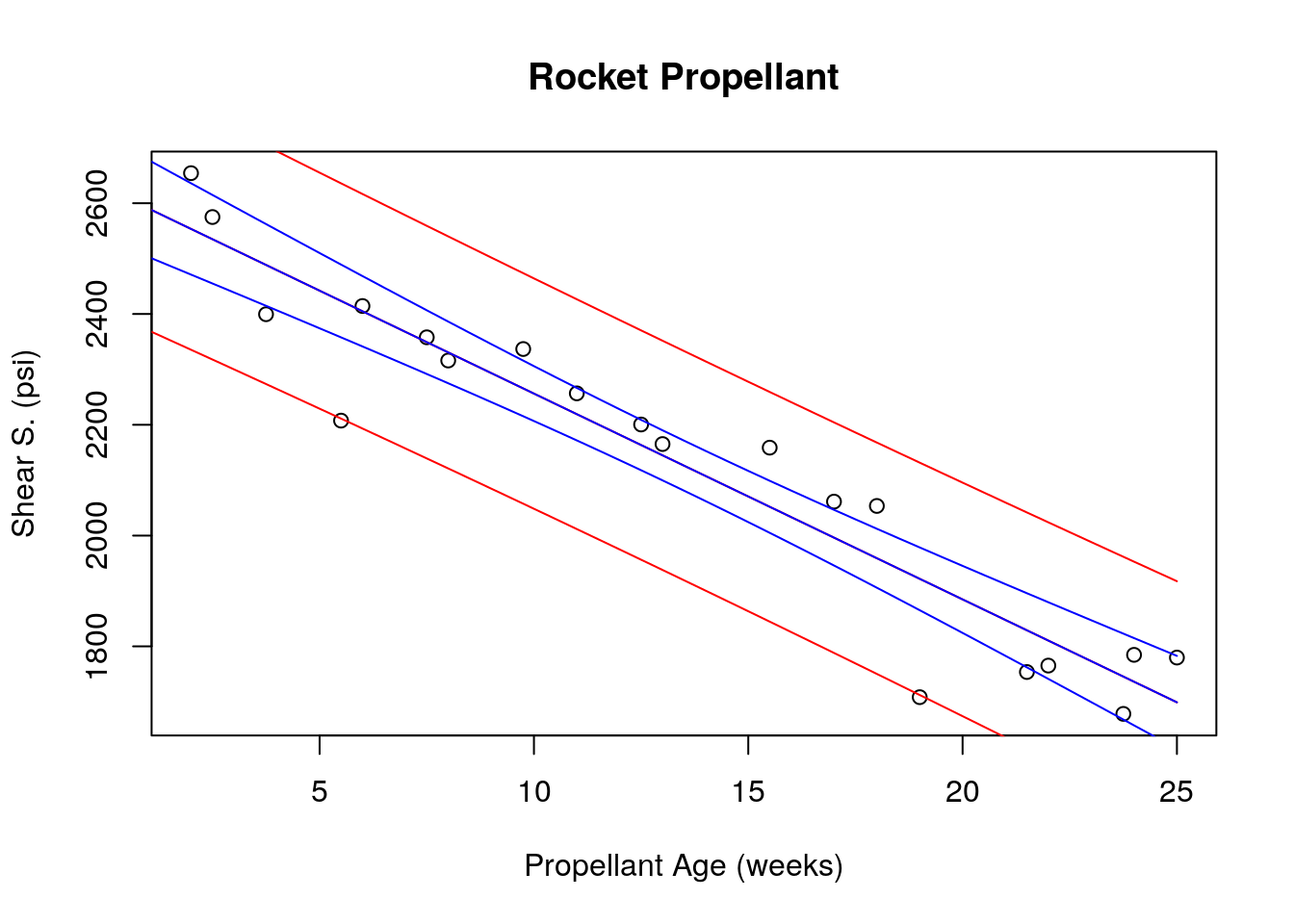NOTE: For your homework download and use the template (https://math.dartmouth.edu/~m50f17/HW2.Rmd)

Read the green comments in the rmd file to see where your answers should go.

## Question-1 (Sample)

Given a fixed confidence interval percentage (say 95%) at what value of x does CI on the mean response achieve its minimum width?

Write an R-chunk using the propellant data which computes the following.

1. Fit a simple linear regression model relating shear strength to age.
2. Plot scatter diagram.
3. Plot two curves (in blue color) that traces upper and lower limits of 95% confidence interval on $$E(y|x_0)$$
4. Plot two curves (in red color) that traces upper and lower limits of 95% prediction interval for $$y$$
5. Print the 95% quantile of the corresponding t distribution

The width of the interval is $2 t_{\alpha/2,n-2} \sqrt{MS_{Res} ((1/n) + (x_0 - \bar{x})^2 / S_{xx} }$ and all terms inside the square root are positive. Therefore it is minimized when $$x_0=\bar{x}$$.

# Computation part of the answer :

shearS<-prop$ShearS age<-prop$Age

plot(age, shearS, xlab = "Propellant Age (weeks)", ylab = "Shear S. (psi)", main = "Rocket Propellant")
fitted <- lm(shearS ~ age)

ageList <- seq(0,25,0.5)
cList <- predict(fitted, list(age = ageList), int = "c", level = 0.95)
pList <- predict(fitted, list(age = ageList), int = "p", level = 0.95)

matlines(ageList, pList, lty='solid' ,  col = "red")
matlines(ageList, cList, lty = 'solid', col = "blue")# since n=20 we look at the t_18 distribution
wantedQuantile <- qt( 0.95, 18) ;
cat("95% quantile is of t_18 is : ", wantedQuantile ) ; 
## 95% quantile is of t_18 is :  1.734064

## Question-2

Plot the same graph as in Question-1 without using R function predict, but instead directly calculating the interval limits we discussed in class. In particular, what are the limits of 95% confidence interval on $$E(y|x_0)$$?

# Computation part of the answer : 

## Question-3

Load the propellant data and fit a simple linear regression model relating shear strength to age.

1. Test the hypothesis $$\beta_1 = -30$$ using confidence level 97.5%.
2. Calculate the limits of 97.5% confidence interval for $$\beta_0$$ and $$\beta_1$$
3. Is there any relation between the answers you find in part (a) and (b) ?
4. Calculate $$R^2$$

# Computation part of the answer : 

## Question-4

Load the propellant data. This time let us consider a relation between square of shear strength and propellant age.

1. Fit a simple linear regression model relating square of shear strength to age. Plot scatter diagram and fitted line.
2. Using analysis-of-variance test for significance of regression (using the formulas we discussed in class)
3. Use t-test and check significance of regression (using the formulas we discussed in class)
4. Does the regression analysis predict a linear relationship between square of shear strength and propellant age ?

# Computation part of the answer : 

## Question-5

Once again using propellant data fit a simple linear regression model between shear strength and propellant age. Consider the steps that we used to obtain t-test for hypothesis $$\beta_1 = G_1$$, and following similar steps in order to develop a test for $$\beta_1 > G_1$$ instead. Then

1. Test the hypothesis statement $\beta_1 > -50$ with confidence level 99.9%.
2. Find the smallest value $$G_1$$ such that the above hypothesis statement is rejected.
3. Similarly what is the smallest value $$G_0$$ such that the statement “$$\beta_0 > G_0$$ with probability 0.999” is rejected.

# Computation part of the answer :